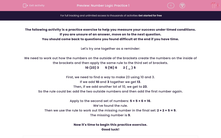# Number Logic Practice 1

In this practice exercise, students will identify a rule which is used to make a number and apply this rule to find a missing number.This content is premium and exclusive to EdPlace subscribers.Key stage:  KS 2

Curriculum topic:   Exam-Style Questions: Verbal Reasoning

Curriculum subtopic:   Exam-Style Questions: Number Logic

Difficulty level:#### Worksheet Overview

If you are unsure of an answer, move on to the next question.

You should come back to questions you found difficult at the end if you have time.

Let's try one together as a reminder:

We need to work out how the numbers on the outside of the brackets create the numbers on the inside of the brackets and then apply the same rule to the third set of brackets.

10 (23) 3        5 (16) 6        2 ( _ ) 5

First, we need to find a way to make 23 using 10 and 3.

If we add 10 and 3 together we get 13.

Then, if we add another lot of 10, we get to 23.

So the rule could be: add the two outside numbers and then add the first number again.

Apply to the second set of numbers: 5 + 5 + 6 = 16.

We’ve found the rule.

Then we use the rule to work out the missing number in the final set: 2 + 2 + 5 = 9.

The missing number is 9.

Now it's time to begin this practice exercise.

Good luck!

### What is EdPlace?

We're your National Curriculum aligned online education content provider helping each child succeed in English, maths and science from year 1 to GCSE. With an EdPlace account you’ll be able to track and measure progress, helping each child achieve their best. We build confidence and attainment by personalising each child’s learning at a level that suits them.

Get started